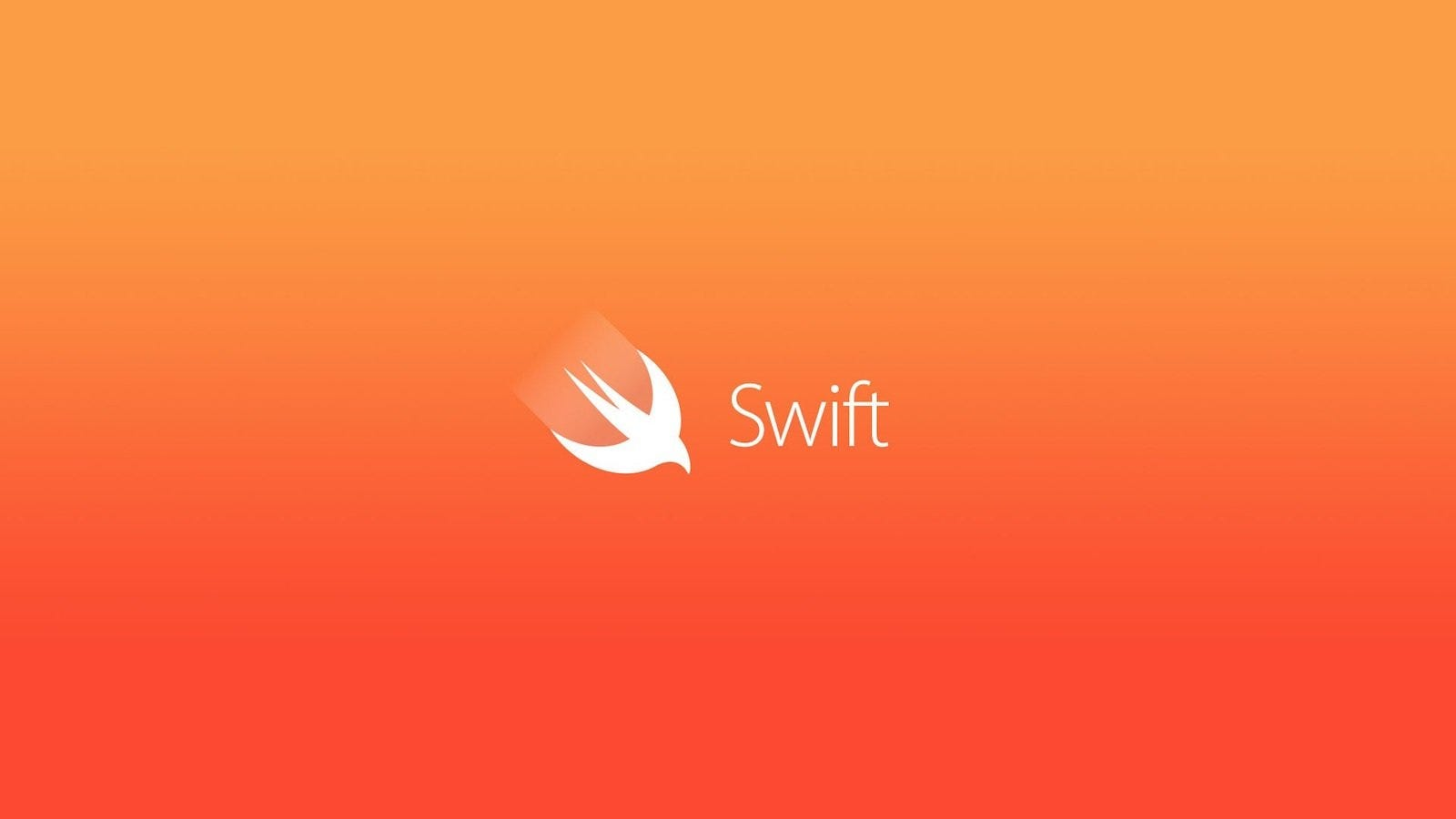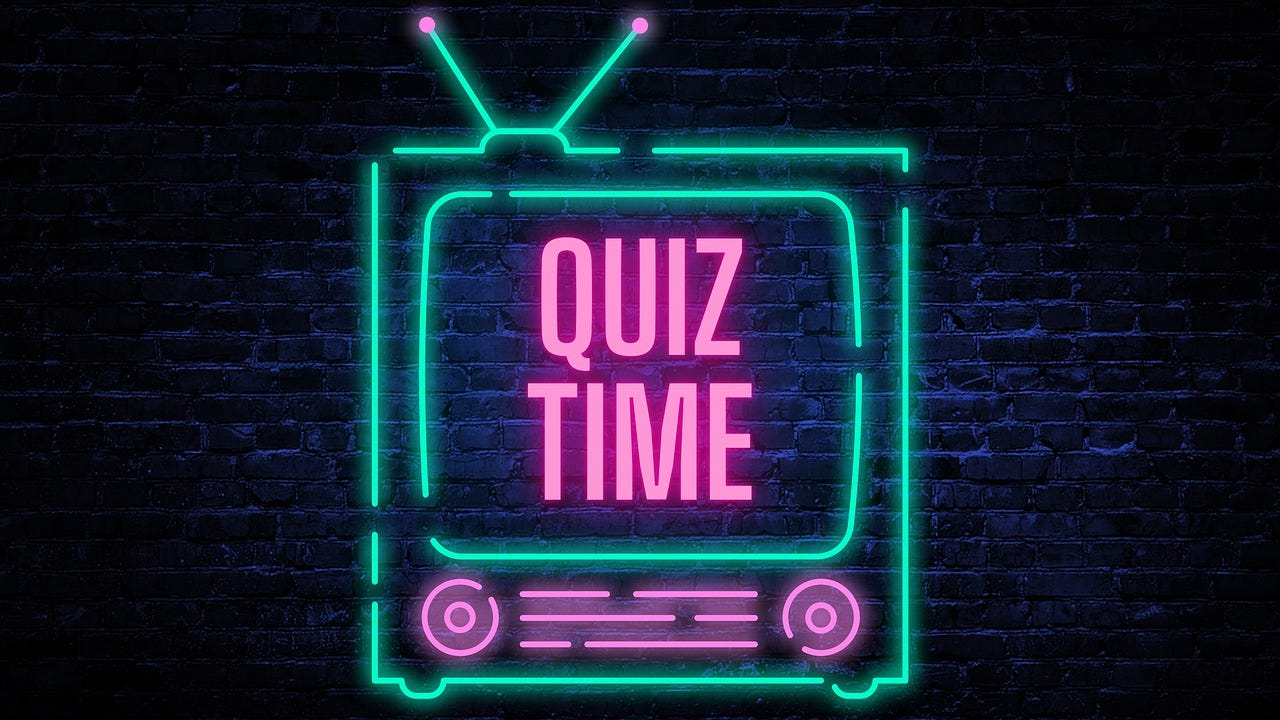# Swift Quiz #02 Fundamentals — Integer, Float, and Boolean

Topics covered: Integer, Int, UInt, Float, Boolean, and Type Alias## Integers:

• Integers are whole numbers without fractional components.

• Swift provides signed (positive, zero, or negative) and unsigned (positive or zero) integers.

• Integers are available in 8, 16, 32, and 64-bit forms, denoted by types like Int8, UInt16, etc.

• You can access the minimum and maximum values of each integer type using min and max properties.

``````    let minValue = UInt8.min  // minValue is equal to 0, and is of type UInt8
let maxValue = UInt8.max  // maxValue is equal to 255, and is of type UInt8
``````

## Int:

• Swift provides a type called “Int”, which has the same size as the platform’s native word size.

• On a 32-bit platform, “Int” is the same size as “Int32”, and on a 64-bit platform, “Int” is the same size as “Int64”.

• Unless you specifically need a certain size of integer, it is recommended to use “Int” for integer values in your code.

``````    let count= 42
// count is inferred to be of type Int
``````

## UInt:

• Swift also provides an unsigned integer type called “UInt”, which has the same size as the platform’s native word size.

• Use “UInt” only when you specifically need an unsigned integer type with the same size as the platform’s native word size. Otherwise, use “Int”.

``````    let positiveNumber: UInt = 10
// positiveNumber is of type UInt
``````

## Floating-Point Numbers:

• Floating-point numbers have a fractional component.

• Swift provides two types for floating-point numbers: Double (64-bit) and Float (32-bit).

• Double has a higher precision than Float, so it is preferred unless specific memory constraints exist.

``````    let pi = 3.14159
// pi is inferred to be of type Double
``````

## Numeric Literals:

• Numeric literals can be expressed in decimal, binary, octal, or hexadecimal formats.

• Numeric literals can contain extra formatting for readability, such as underscores.

``````    let oneMillion = 1_000_000
let decimalDouble = 12.1875
``````

## Booleans:

• Swift provides a Boolean type called Bool, which is used to declare variables or constants that hold boolean values.

• Boolean values are particularly useful for control flow and decision-making in code.

let isRaining = true // isRaining is inferred to be of type Bool

## Type Safety and Type Inference:

• Swift is a type-safe language, meaning it performs type checks during compilation to catch mismatched types.

• Type inference allows the compiler to deduce the type of an expression based on the provided values.

• Swift requires fewer explicit type declarations compared to languages like Objective-C.

``````    let decimalInteger = 17
let binaryInteger = 0b10001  // 17 in binary notation
``````

## Type Aliases:

• Type aliases provide alternative names for existing types.

• They are useful when you want to use a more contextually appropriate name for a type.

``````    typealias AudioSample = UInt16
let maxAmplitudeFound = AudioSample.min
// maxAmplitudeFound is equal to 0, and is of type UInt16
``````

## QuizzesQuiz 1:

What is the maximum value that can be stored in an Int8 in Swift?

a) 127

b) 255

c) 32767

d) -128

Int8 is a signed 8-bit integer type in Swift, which means it can store values from -128 to 127. The maximum value it can hold is 127.

Quiz 2:

Which type should be used in Swift to store a large floating-point number with high precision?

a) Float

b) Double

c) Decimal

d) Int

Explanation: Swift provides two types for floating-point numbers: Float (32-bit) and Double (64-bit). Double has a higher precision than Float, so it is preferred when high precision is required.

Quiz 3:

What is the output of the following code snippet?

``````let number: Int = 42
let result = number % 7
print(result)
``````

a) 42

b) 0

c) 6

d) 7

Explanation: The code snippet calculates the remainder when the variable “number” (which is 42) is divided by 7 using the modulo operator (%). The remainder of 42 divided by 7 is 6, so the output is 6.

Quiz 4:

Which of the following is a valid type alias declaration in Swift?

a) typealias Int8 = UInt8

b) typealias String = Int

c) typealias Float = Double

d) typealias Bool = Int

Answer: a) typealias Int8 = UInt8

Explanation: Type aliases provide alternative names for existing types. In the given options, typealias Int8 = UInt8 is a valid declaration that creates an alias Int8 for the UInt8 type.

Quiz 5:

What is the size of the Int type in Swift on a 64-bit platform?

a) 8-bit

b) 16-bit

c) 32-bit

d) 64-bit

Explanation: The Int type in Swift has the same size as the platform’s native word size. On a 64-bit platform, Int is a 64-bit integer type.

Quiz 6:

Which of the following is an example of a hexadecimal numeric literal in Swift?

a) 0b10001

b) 17

c) 0x11

d) 12.1875

Explanation:

In Swift Hexadecimal numeric literals are represented with the prefix “0x”. In the given options, 0x11 is a hexadecimal literal representing the decimal value 17.

Quiz 7:

What is the type of the variable maxAmplitudeFound in the following code?

``````typealias AudioSample = UInt16
let maxAmplitudeFound = AudioSample.min
``````

a) Int

b) UInt8

c) Float

d) UInt16

Explanation: The code snippet declares a typealias AudioSample for UInt16 and assigns the minimum value of UInt16 to the variable maxAmplitudeFound. Therefore, maxAmplitudeFound is of type UInt16.

Quiz 8:

Which of the following is the correct way to access the maximum value of an Int32 in Swift?

a) Int32.maxValue

b) Int32.maximum

c) Int32.max

d) Int32.maximumValue

Explanation: In Swift, to access the maximum value of an integer type, the convention is to use the type name followed by “.max”. Therefore, Int32.max is the correct way to access the maximum value of an Int32.

Quiz 9:

What is the output of the following code snippet?

``````let x: Double = 1.5
let y: Int = 2
let result = x + Double(y)
print(result)
``````

a) 3.5

b) 4.0

c) 3

d) Error

Explanation: The code snippet adds a Double value (1.5) to an Int value (2) after converting the Int value to Double. The result of the addition is 3.5, which is a Double value, so the output is 3.5.

Quiz 10:

Which of the following is the correct way to declare a variable in Swift without assigning an initial value?

a) var number: Int

b) var number: Int = nil

c) let number: Int

d) let number: Int = nil